图像旋转_图像旋转识别 - CSDN

• 图像旋转的原理与实现 一般图像旋转是以图像的中心为原点，旋转一定的角度，也就是将图像上的所有像素都旋转一个相同的角度。旋转图像的的大小一般会改变，即可以把转出显示区域的图像截去，或者扩大图像范围来...
图像旋转的原理与实现

一般图像的旋转是以图像的中心为原点，旋转一定的角度，也就是将图像上的所有像素都旋转一个相同的角度。旋转后图像的的大小一般会改变，即可以把转出显示区域的图像截去，或者扩大图像范围来显示所有的图像。图像的旋转变换也可以用矩阵变换来表示。设点逆时针旋转角后的对应点为。那么，旋转前后点、的坐标分别是：(3-6)(3-7)

写成矩阵表达式为(3-8)

其逆运算为(3-9)

利用上述方法进行图像旋转时需要注意如下两点：

（1）图像旋转之前，为了避免信息的丢失，一定要有坐标平移。

（2）图像旋转之后，会出现许多空洞点。对这些空洞点必须进行填充处理，否则画面效果不好，一般也称这种操作为插值处理。

以上所讨论的旋转是绕坐标轴原点(0,0)进行的。如果图像旋转是绕一个指定点(a,b)旋转，则先要将坐标系平移到该点，再进行旋转，然后将旋转后的图象平移回原来的坐标原点，这实际上是图像的复合变换。如将一幅图像绕点(a,b)逆时针旋转度，首先将原点平移到(a,b)，即(3-10)

然后旋转(3-11)

然后再平移回来(3-12)

综上所述，变换矩阵为。

展开全文C++
• 给定一个 n × n 的二维矩阵表示一个图像。 将图像顺时针旋转 90 度。 说明： 你必须在原地旋转图像，这意味着你需要直接修改输入的二维矩阵。请不要使用另一个矩阵来旋转图像。 示例 1: 给定 matrix = [ ...
给定一个 n × n 的二维矩阵表示一个图像。
将图像顺时针旋转 90 度。
说明：
你必须在原地旋转图像，这意味着你需要直接修改输入的二维矩阵。请不要使用另一个矩阵来旋转图像。
示例 1:
给定 matrix =
[
[1,2,3],
[4,5,6],
[7,8,9]
],

原地旋转输入矩阵，使其变为:
[
[7,4,1],
[8,5,2],
[9,6,3]
]

示例 2:
给定 matrix =
[
[ 5, 1, 9,11],
[ 2, 4, 8,10],
[13, 3, 6, 7],
[15,14,12,16]
],

原地旋转输入矩阵，使其变为:
[
[15,13, 2, 5],
[14, 3, 4, 1],
[12, 6, 8, 9],
[16, 7,10,11]
]


本题要求不能开辟新空间，仅仅是原地旋转，所以不可以对于一个元素找到其新位置直接过去，在一个二维数组中原地旋转，只能要求数组内的元素两两交换（这样才不会覆盖目标位置上原来的数据）来达到旋转的目的。
观察，对于一个3*3数组，仅仅需要两轮循环，每次循环都是将四个角上的元素进行两两交换，且存下最左端的元素，将四个元素依次进行顺时针交换。


class Solution {
public void rotate(int[][] matrix) {
if(matrix == null)
return;

int n = matrix.length;

for(int i = 0; i < n/2; i++){
for(int j = i; j < n-1-i; j++){
int temp = matrix[i][j];
matrix[i][j] = matrix[n-1-j][i];
matrix[n-1-j][i] = matrix[n-1-i][n-1-j];
matrix[n-1-i][n-1-j] = matrix[j][n-1-i];
matrix[j][n-1-i] = temp;
}
}

}
}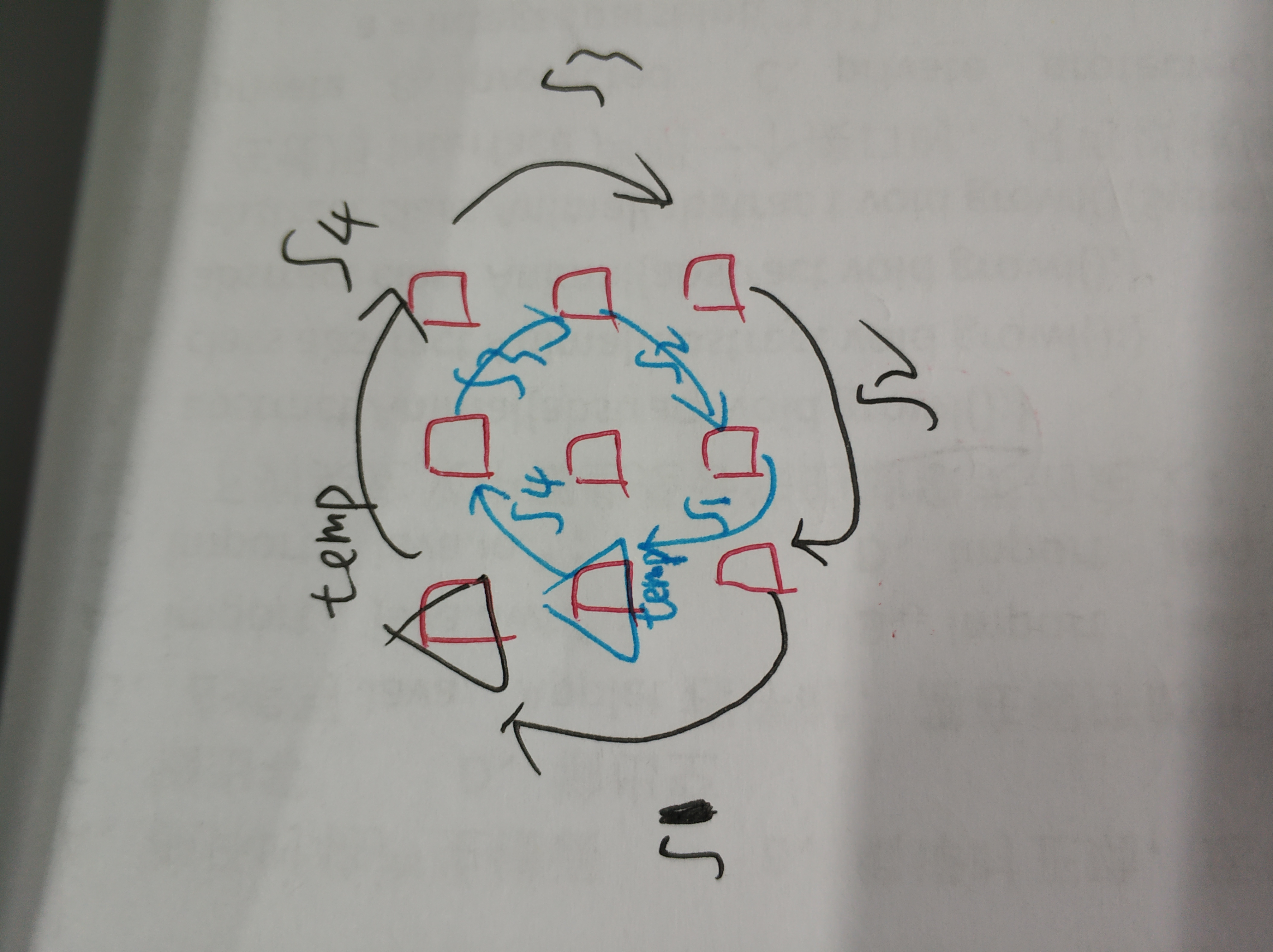展开全文• ## 图像旋转原理及实现

万次阅读 多人点赞 2018-10-25 16:10:15
在一次数字图像处理课中，我接触到了图片旋转的一些原理，当时没完全想明白，课后通过一段时间的学习，终于完成了图片旋转的matlab程序。开始觉得应该挺简单的，但终究是纸上谈兵，在实现的过程中遇到很多问题。
建议阅读该博客的朋友最好对插值、matlab编程、数字图像有一些了解，另外所有代码和测试图片都可以到GitHub去下载。
在一次数字图像处理课中，我接触到了图片旋转的一些原理，当时没完全想明白，课后通过一段时间的学习，终于完成了图片旋转的matlab程序。开始觉得应该挺简单的，但终究是纸上谈兵，在实现的过程中遇到很多问题。
1 旋转矩形
首先建议阅读图像旋转算法原理-旋转矩阵，这篇博客可以让你很好地理解图像中的每一个点是如何进行旋转操作的。其中涉及到了图像原点与笛卡尔坐标原点之间的相互转换以及点旋转的一些公式推导。
我们一般认为图像应该绕着中心点旋转，但是图像的原点在左上角，在计算的时候首先需要将左上角的原点移到图像中心，并且Y轴需要翻转。设一点(X0,Y0)(X_0,Y_0)，图像宽为WW，高为HH，原点变换后的点为(X1,Y1)(X_1,Y_1)，变换如下式。
(1)[X1Y11]=[X0Y01][1000−10−0.5W0.5H1]
\begin{bmatrix}
X_1 &amp; Y_1 &amp; 1
\end{bmatrix} =
\begin{bmatrix}
X_0 &amp; Y_0 &amp; 1
\end{bmatrix}
\begin{bmatrix}
1 &amp; 0 &amp; 0 \\
0 &amp; -1 &amp; 0 \\
-0.5W &amp; 0.5H &amp; 1
\end{bmatrix} \tag{1}

图像旋转角度为θ\theta，设原点变换后通过旋转矩阵旋转θ\theta后的点为(X2,Y2)(X_2,Y_2)，公式如下。
(2)[X2Y21]=[X1Y11][cosθ−sinθ0sinθcosθ0001]
\begin{bmatrix}
X_2 &amp; Y_2 &amp; 1
\end{bmatrix} =
\begin{bmatrix}
X_1 &amp; Y_1 &amp; 1
\end{bmatrix}
\begin{bmatrix}
cos\theta &amp; -sin \theta &amp; 0 \\
sin\theta &amp; cos\theta &amp; 0 \\
0 &amp; 0 &amp; 1
\end{bmatrix} \tag{2}

旋转后的图像的宽为W&quot;W^&quot;，高为H&quot;H^&quot;，那么从笛卡尔坐标原点变换回左上角的公式如下。
(3)[X3Y31]=[X2Y21][1000−100.5W&quot;0.5H&quot;1]
\begin{bmatrix}
X_3 &amp; Y_3 &amp; 1
\end{bmatrix} =
\begin{bmatrix}
X_2 &amp; Y_2 &amp; 1
\end{bmatrix}
\begin{bmatrix}
1 &amp; 0 &amp; 0 \\
0 &amp; -1 &amp; 0 \\
0.5W^&quot; &amp; 0.5H^&quot; &amp; 1
\end{bmatrix} \tag{3}

综上可得，原图像的一点(X0,Y0)(X_0,Y_0)经过如下公式可以变换到旋转后的(X3,Y3)(X_3,Y_3)。
(4)[X3Y31]=[X0Y01]∗[1000−10−0.5W0.5H1][cosθ−sinθ0sinθcosθ0001][1000−100.5W&quot;0.5H&quot;1]
\begin{bmatrix}
X_3 &amp; Y_3 &amp; 1
\end{bmatrix} =
\begin{bmatrix}
X_0 &amp; Y_0 &amp; 1
\end{bmatrix} *
\begin{bmatrix}
1 &amp; 0 &amp; 0 \\
0 &amp; -1 &amp; 0 \\
-0.5W &amp; 0.5H &amp; 1
\end{bmatrix}
\begin{bmatrix}
cos\theta &amp; -sin \theta &amp; 0 \\
sin\theta &amp; cos\theta &amp; 0 \\
0 &amp; 0 &amp; 1
\end{bmatrix}
\begin{bmatrix}
1 &amp; 0 &amp; 0 \\
0 &amp; -1 &amp; 0 \\
0.5W^&quot; &amp; 0.5H^&quot; &amp; 1
\end{bmatrix} \tag{4}

同理，旋转后的一点(X3,Y3)(X_3,Y_3)经过如下公式可以变换回原图像的(X0,Y0)(X_0,Y_0)。
(5)[X0Y01]=[X3Y31]∗[1000−10−0.5W&quot;0.5H&quot;1][cosθsinθ0−sinθcosθ0001][1000−100.5W0.5H1]
\begin{bmatrix}
X_0 &amp; Y_0 &amp; 1
\end{bmatrix} =
\begin{bmatrix}
X_3 &amp; Y_3 &amp; 1
\end{bmatrix} *
\begin{bmatrix}
1 &amp; 0 &amp; 0 \\
0 &amp; -1 &amp; 0 \\
-0.5W^&quot; &amp; 0.5H^&quot; &amp; 1
\end{bmatrix}
\begin{bmatrix}
cos\theta &amp; sin \theta &amp; 0 \\
-sin\theta &amp; cos\theta &amp; 0 \\
0 &amp; 0 &amp; 1
\end{bmatrix}
\begin{bmatrix}
1 &amp; 0 &amp; 0 \\
0 &amp; -1 &amp; 0 \\
0.5W &amp; 0.5H &amp; 1
\end{bmatrix} \tag{5}

在开始下一部分之前，先要得到旋转后图像的大小，设原图像高为m，宽为n。代码如下，虽然看起来有点复杂，建议朋友们自己画张图看一下，那样会一目了然。
% image size after rotation
[m, n, o] = size(img);
new_m = ceil(abs(m*cosd(degree)) + abs(n*sind(degree)));
new_n = ceil(abs(n*cosd(degree)) + abs(m*sind(degree)));

2 前向映射
建议阅读图像旋转变换-matlab实现，里面采用的是前向映射的方法。
什么是前向映射呢？就是通过原图像的坐标计算旋转之后的坐标，并将相应的灰度值传给旋转后的图像。这样遇到最大的问题就是出现了一些有规律的噪声，如下图。通过代码来分析一下产生这种现象的原因，前向映射的代码如下。m1，m2和m3这三个矩阵可以对应到公式(4)中的三个矩阵，可以看出转换后的坐标是小数，并且进行了取整操作，这样部分像素并没有对应的灰度值。
% forward mapping matrices
m1 = [1 0 0; 0 -1 0; -0.5*n 0.5*m 1];
m2 = [cosd(degree) -sind(degree) 0; sind(degree) cosd(degree) 0; 0 0 1];
m3 = [1 0 0; 0 -1 0; 0.5*new_n 0.5*new_m 1];

for i = 1:n
for j = 1:m
new_coordinate = [i j 1]*m1*m2*m3;
col = round(new_coordinate(1));
row = round(new_coordinate(2));
% no-rotation image's coordinates to rotated image's coordinates
new_img_forward(row, col, 1) = img(j, i, 1);
new_img_forward(row, col, 2) = img(j, i, 2);
new_img_forward(row, col, 3) = img(j, i, 3);
end
end

3 反向映射
为了解决上述问题，可以采用反向映射的方法，即从旋转后的图像出发，找到对应的原图像的点，然后将原图像中的灰度值传递过来即可，这样旋转后的图像的每个像素肯定可以对应到原图像中的一个点，采取不同策略可以让像素对应地更加准确，建议首先阅读图像旋转算法与实现以及基于Matlab的双线性插值算法在图像旋转中的应用来更好的理解反向映射。
下图可以很好地理解反向映射的过程。下面代码实现了最近邻插值和双线性插值，其中rm1，rm2和rm3表示式(5)中的三个转换矩阵。
% reverse mapping matrices
rm1 = [1 0 0; 0 -1 0; -0.5*new_n 0.5*new_m 1];
rm2 = [cosd(degree) sind(degree) 0; -sind(degree) cosd(degree) 0; 0 0 1];
rm3 = [1 0 0; 0 -1 0; 0.5*n 0.5*m 1];

for i = 1:new_n
for j = 1:new_m
% rotated image's coordinates to no-rotation image's coordinates
old_coordinate = [i j 1]*rm1*rm2*rm3;
col = round(old_coordinate(1));
row = round(old_coordinate(2));
% prevent border overflow
if row < 1 || col < 1 || row > m || col > n
new_img_nnp(j, i) = 0;
new_img_lp(j, i) = 0;
else
% nearest neighbor interpolation
new_img_nnp(j, i, 1) = img(row, col, 1);
new_img_nnp(j, i, 2) = img(row, col, 2);
new_img_nnp(j, i, 3) = img(row, col, 3);

% bilinear interpolation
left = floor(col);
right = ceil(col);
top = floor(row);
bottom = ceil(row);

a = col - left;
b = row - top;
new_img_lp(j, i, 1) = (1-a)*(1-b)*img(top, left, 1) + a*(1-b)*img(top, right, 1) + ...
(1-a)*b*img(bottom, left, 1) + a*b*img(bottom, right, 1);
new_img_lp(j, i, 2) = (1-a)*(1-b)*img(top, left, 2) + a*(1-b)*img(top, right, 2) + ...
(1-a)*b*img(bottom, left, 2) + a*b*img(bottom, right, 2);
new_img_lp(j, i, 3) = (1-a)*(1-b)*img(top, left, 3) + a*(1-b)*img(top, right, 3) + ...
(1-a)*b*img(bottom, left, 2) + a*b*img(bottom, right, 3);
end
end
end

经过反向映射得到如下结果，下面展示的是反向映射+双线性插值的结果，很好的解决了前向映射出现有规律噪声的问题。4 总结
文章介绍了关于图像旋转的一些原理和相关的实现，从旋转矩阵开始，到前向映射，再到反向映射，反向映射中采用了最近邻插值和双线性插值，较好的实现了图片的旋转功能。建议朋友们多阅读文中引用的一些博客和论文，这样对图像旋转的很多细节会有更好的把握。
http://blog.csdn.net/liyuan02/article/details/6750828
http://blog.csdn.net/abee23/article/details/7398749
http://www.cnblogs.com/mlv5/archive/2012/02/02/2336321.html
http://www.cqvip.com/qk/80356a/201004/35811191.html

展开全文图像旋转 matlab 数字图像
• 该系列文章是讲解Python OpenCV图像处理知识，前期主要讲解图像入门、OpenCV基础用法，中期讲解图像处理的各种算法，包括图像锐化算子、图像增强技术、图像分割等，后期结合深度学习研究图像识别、图像分类应用。...
该系列文章是讲解Python OpenCV图像处理知识，前期主要讲解图像入门、OpenCV基础用法，中期讲解图像处理的各种算法，包括图像锐化算子、图像增强技术、图像分割等，后期结合深度学习研究图像识别、图像分类应用。希望文章对您有所帮助，如果有不足之处，还请海涵~
该系列在github所有源代码：https://github.com/eastmountyxz/ImageProcessing-Python
PS：请求帮忙点个Star，哈哈，第一次使用Github，以后会分享更多代码，一起加油。
同时推荐作者的C++图像系列知识：
[数字图像处理] 一.MFC详解显示BMP格式图片
[数字图像处理] 二.MFC单文档分割窗口显示图片
[数字图像处理] 三.MFC实现图像灰度、采样和量化功能详解
[数字图像处理] 四.MFC对话框绘制灰度直方图
[数字图像处理] 五.MFC图像点运算之灰度线性变化、灰度非线性变化、阈值化和均衡化处理详解
[数字图像处理] 六.MFC空间几何变换之图像平移、镜像、旋转、缩放详解
[数字图像处理] 七.MFC图像增强之图像普通平滑、高斯平滑、Laplacian、Sobel、Prewitt锐化详解
前文参考：
[Python图像处理] 一.图像处理基础知识及OpenCV入门函数
[Python图像处理] 二.OpenCV+Numpy库读取与修改像素
[Python图像处理] 三.获取图像属性、兴趣ROI区域及通道处理
[Python图像处理] 四.图像平滑之均值滤波、方框滤波、高斯滤波及中值滤波
[Python图像处理] 五.图像融合、加法运算及图像类型转换
本篇文章主要讲解Python调用OpenCV实现图像位移操作、旋转和翻转效果，包括四部分知识：图像缩放、图像旋转、图像翻转、图像平移。全文均是基础知识，希望对您有所帮助。
1.图像缩放
2.图像旋转
3.图像翻转
4.图像平移
PS：文章参考自己以前系列图像处理文章及OpenCV库函数，同时部分参考网易云视频，推荐大家去学习。同时，本篇文章涉及到《计算机图形学》基础知识，请大家下来补充。
推荐原理文章：OpenCV2:图像的几何变换,平移、镜像、缩放、旋转
投票闲谈：
最后希望大家帮我2019年CSDN博客之星投投票，每天可以投5票喔，截止时间2020年2月19日，谢谢大家！八年，在CSDN分享了410篇文章，15个专栏，400多万人次浏览，包括Python人工智能、数据挖掘、网络爬虫、图象处理、网络安全、JAVA网站、Android开发、LAMP/WAMP、C#网络编程、C++游戏、算法和数据结构、面试总结、人生感悟等。当然还有我和你的故事，感恩一路有你，感谢一路同行，希望通过编程分享帮助到更多人，也希望学成之后回贵州教更多学生。因为喜欢，所以分享，且看且珍惜，加油！等我四年学成归来～
投票地址：http://m234140.nofollow.ax.mvote.cn/opage/ed8141a0-ed19-774b-6b0d-39c3aaf89dde.html?from=singlemessage一.图像缩放
图像缩放主要调用resize()函数实现，具体如下：
result = cv2.resize(src, dsize[, result[. fx[, fy[, interpolation]]]])
其中src表示原始图像，dsize表示缩放大小，fx和fy也可以表示缩放大小倍数，他们两个（dsize或fx\fy）设置一个即可实现图像缩放。例如：

result = cv2.resize(src, (160,160))
result = cv2.resize(src, None, fx=0.5, fy=0.5)

图像缩放：设（x0, y0）是缩放后的坐标，（x, y）是缩放前的坐标，sx、sy为缩放因子，则公式如下：
$\left[ \begin{matrix} x_0 & y_0 & 1 \end{matrix} \right] =\left[ \begin{matrix} x & y & 1 \end{matrix} \right] \left[ \begin{matrix} sx & 0 & 0 \\ 0 & sy & 0 \\ 0 & 0 & 1 \end{matrix} \right] \tag{1}$
代码示例如下所示：
#encoding:utf-8
import cv2
import numpy as np

#读取图片

#图像缩放
result = cv2.resize(src, (200,100))
print result.shape

#显示图像
cv2.imshow("src", src)
cv2.imshow("result", result)

#等待显示
cv2.waitKey(0)
cv2.destroyAllWindows()

输出结果如下图所示，图像缩小为（200，100）像素。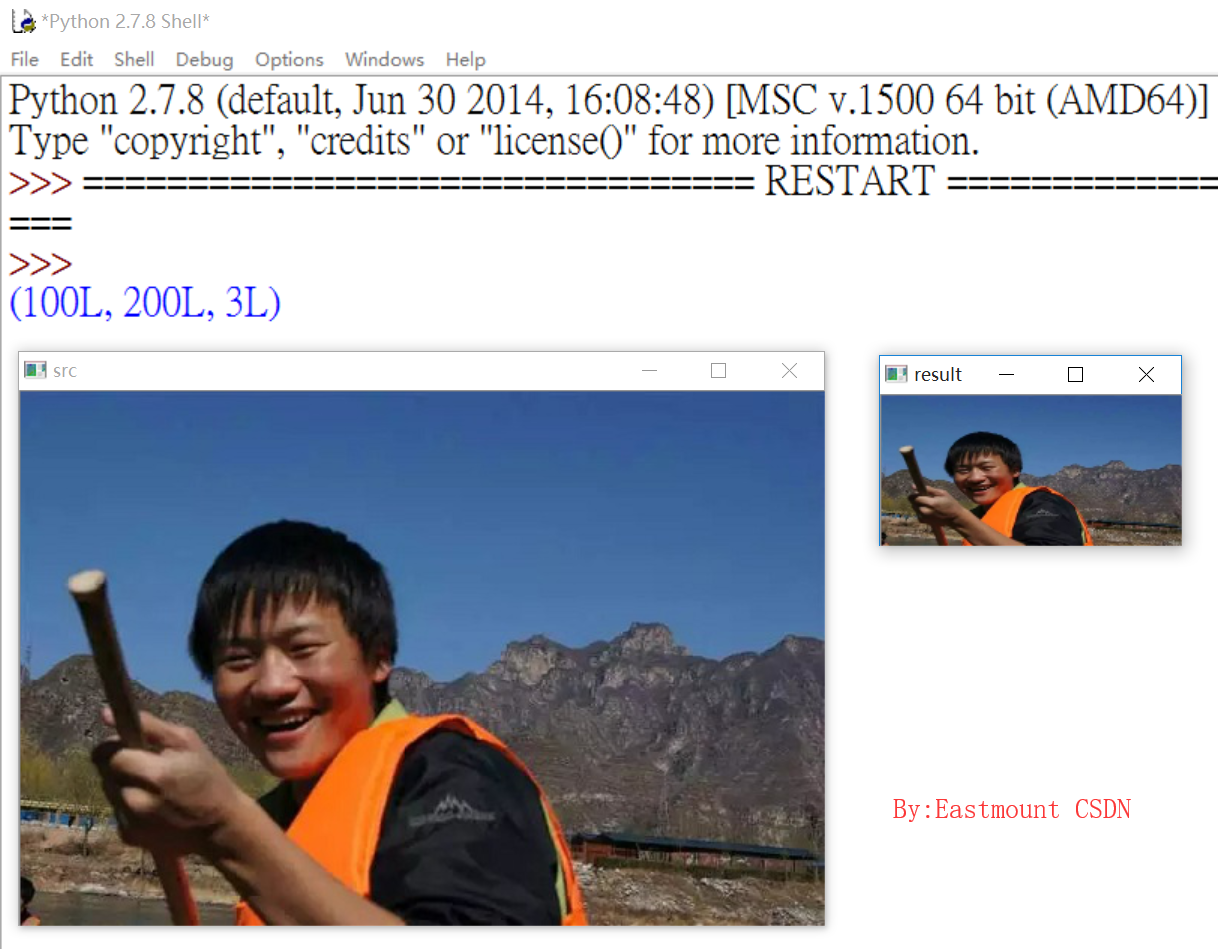需要注意的是，代码中 cv2.resize(src, (200,100)) 设置的dsize是列数为200，行数为100。
同样，可以获取原始图像像素再乘以缩放系数进行图像变换，代码如下所示。
#encoding:utf-8
import cv2
import numpy as np

#读取图片
rows, cols = src.shape[:2]
print rows, cols

#图像缩放 dsize(列,行)
result = cv2.resize(src, (int(cols*0.6), int(rows*1.2)))

#显示图像
cv2.imshow("src", src)
cv2.imshow("result", result)

#等待显示
cv2.waitKey(0)
cv2.destroyAllWindows()

输出结果如下图所示：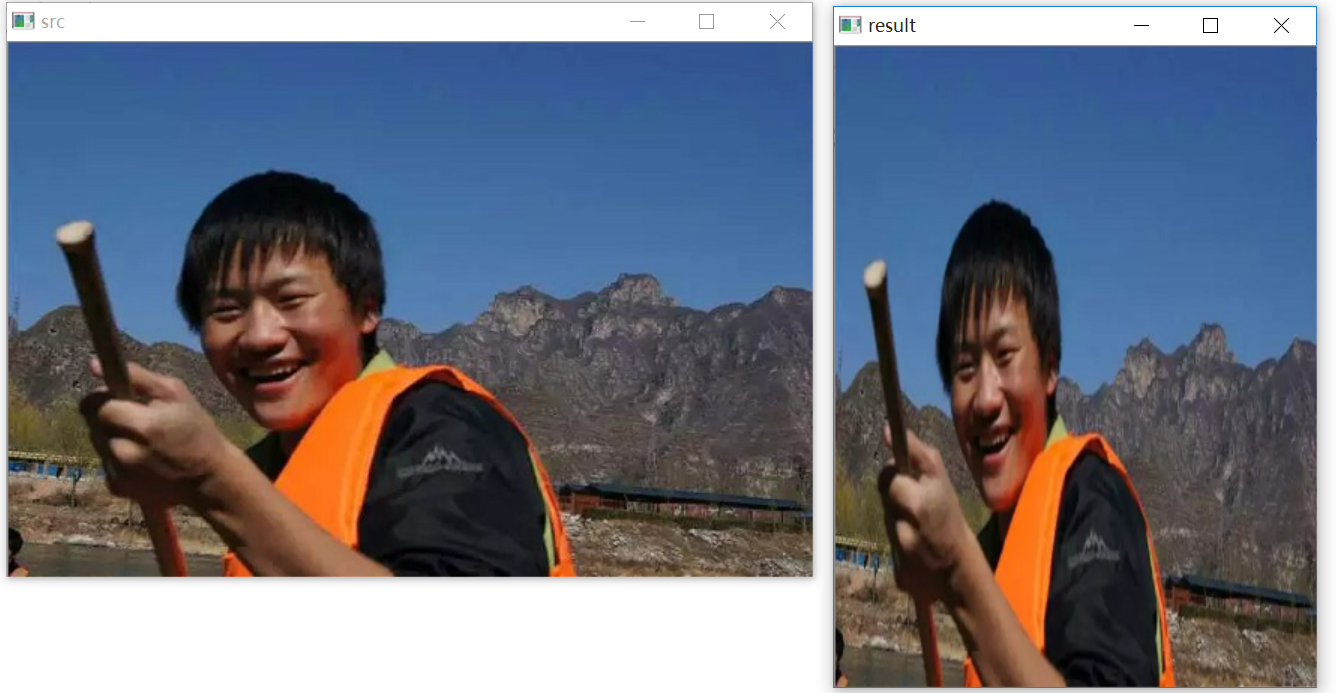最后讲解(fx,fy)缩放倍数的方法对图像进行放大或缩小。
#encoding:utf-8
import cv2
import numpy as np

#读取图片
rows, cols = src.shape[:2]
print rows, cols

#图像缩放
result = cv2.resize(src, None, fx=0.3, fy=0.3)

#显示图像
cv2.imshow("src", src)
cv2.imshow("result", result)

#等待显示
cv2.waitKey(0)
cv2.destroyAllWindows()

最后输出的结果如下图所示，这是按例比0.3*0.3缩小的。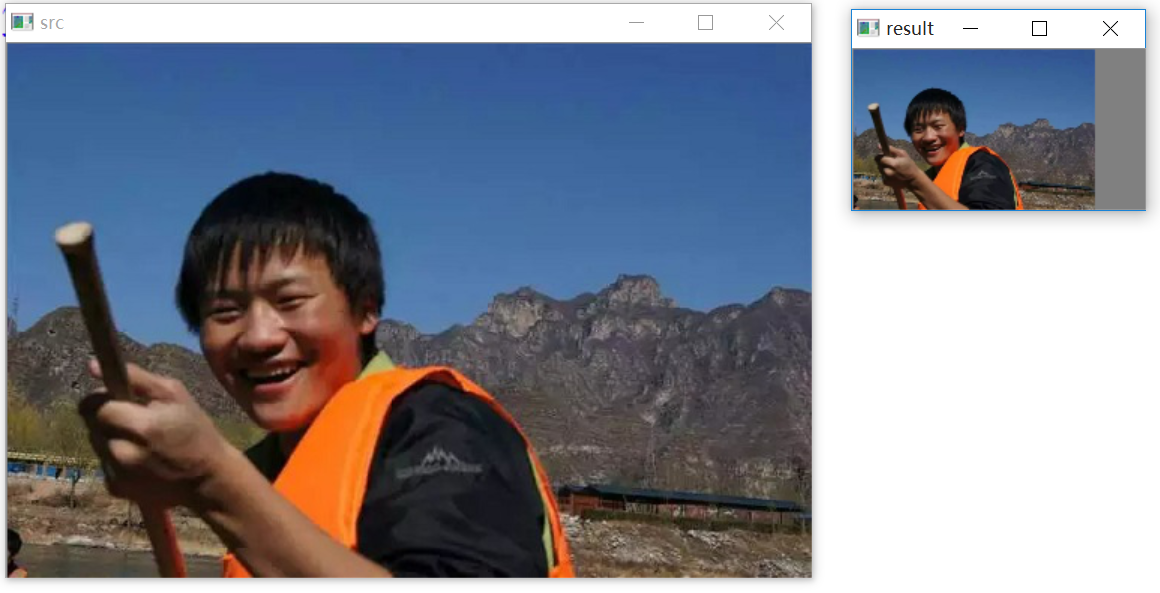二、图像旋转
图像旋转主要调用getRotationMatrix2D()函数和warpAffine()函数实现，绕图像的中心旋转，具体如下：

M = cv2.getRotationMatrix2D((cols/2, rows/2), 30, 1)
参数分别为：旋转中心、旋转度数、scale
rotated = cv2.warpAffine(src, M, (cols, rows))
参数分别为：原始图像、旋转参数、原始图像宽高

图像旋转：设（x0, y0）是旋转后的坐标，（x, y）是旋转前的坐标，(m,n)是旋转中心，a是旋转的角度，(left,top)是旋转后图像的左上角坐标，则公式如下：
$\left[ \begin{matrix} x_0 & y_0 & 1 \end{matrix} \right] = \left[ \begin{matrix} x & y & 1 \end{matrix} \right] \left[ \begin{matrix} 1 & 0 & 0 \\ 0 & -1 & 0 \\ -m & n & 1 \end{matrix} \right] \left[ \begin{matrix} cosa & -sina & 0 \\ sina & cosa & 0 \\ 0 & 0 & 1 \end{matrix} \right] \left[ \begin{matrix} 1 & 0 & 0 \\ 0 & -1 & 0 \\ left & top & 1 \end{matrix} \right] \tag{1}$
代码如下所示：
#encoding:utf-8
import cv2
import numpy as np

#读取图片

#原图的高、宽 以及通道数
rows, cols, channel = src.shape

#绕图像的中心旋转
#参数：旋转中心 旋转度数 scale
M = cv2.getRotationMatrix2D((cols/2, rows/2), 30, 1)
#参数：原始图像 旋转参数 元素图像宽高
rotated = cv2.warpAffine(src, M, (cols, rows))

#显示图像
cv2.imshow("src", src)
cv2.imshow("rotated", rotated)

#等待显示
cv2.waitKey(0)
cv2.destroyAllWindows()

输出结果如下图所示：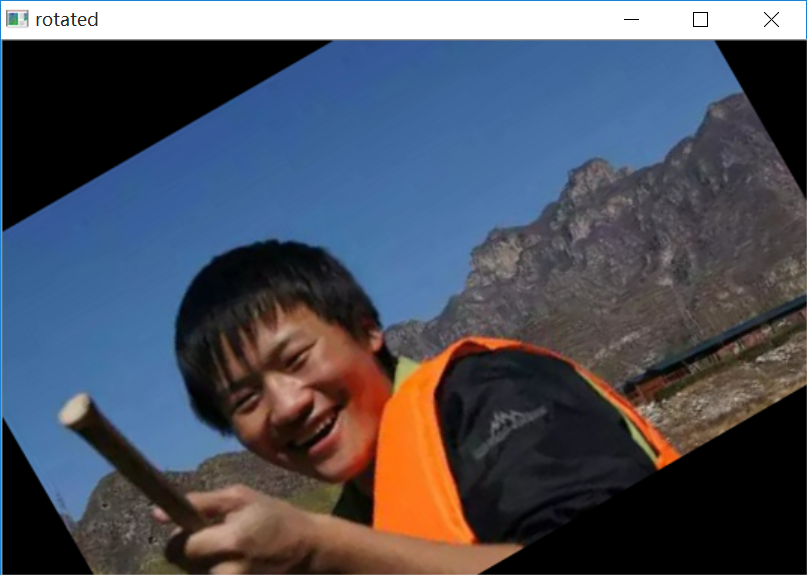如果设置-90度，则核心代码和图像如下所示。
M = cv2.getRotationMatrix2D((cols/2, rows/2), -90, 1)
rotated = cv2.warpAffine(src, M, (cols, rows))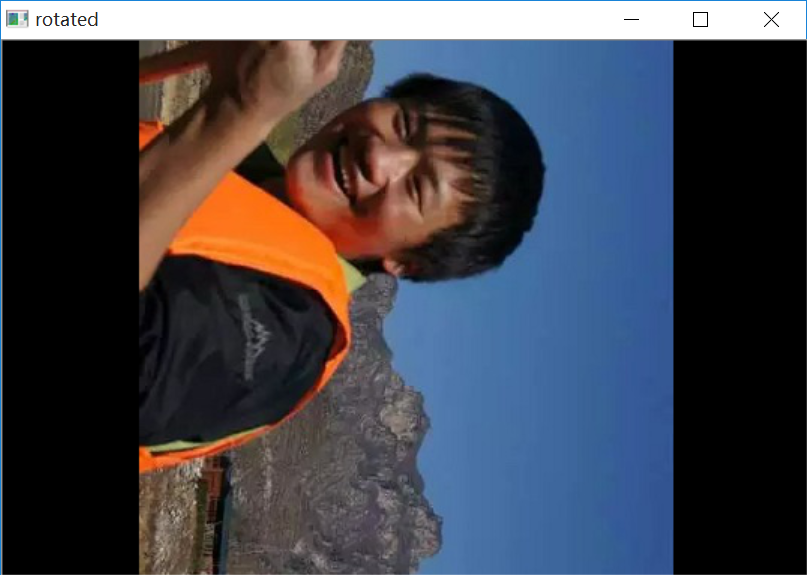三、图像翻转
图像翻转在OpenCV中调用函数flip()实现，原型如下：
dst = cv2.flip(src, flipCode)
其中src表示原始图像，flipCode表示翻转方向，如果flipCode为0，则以X轴为对称轴翻转，如果fliipCode>0则以Y轴为对称轴翻转，如果flipCode<0则在X轴、Y轴方向同时翻转。
代码如下所示：
#encoding:utf-8
import cv2
import numpy as np
import matplotlib.pyplot as plt

#读取图片
src = cv2.cvtColor(img,cv2.COLOR_BGR2RGB)

#图像翻转
#0以X轴为对称轴翻转 >0以Y轴为对称轴翻转 <0X轴Y轴翻转
img1 = cv2.flip(src, 0)
img2 = cv2.flip(src, 1)
img3 = cv2.flip(src, -1)

#显示图形
titles = ['Source', 'Image1', 'Image2', 'Image3']
images = [src, img1, img2, img3]
for i in xrange(4):
plt.subplot(2,2,i+1), plt.imshow(images[i], 'gray')
plt.title(titles[i])
plt.xticks([]),plt.yticks([])
plt.show()

输出结果如下图所示：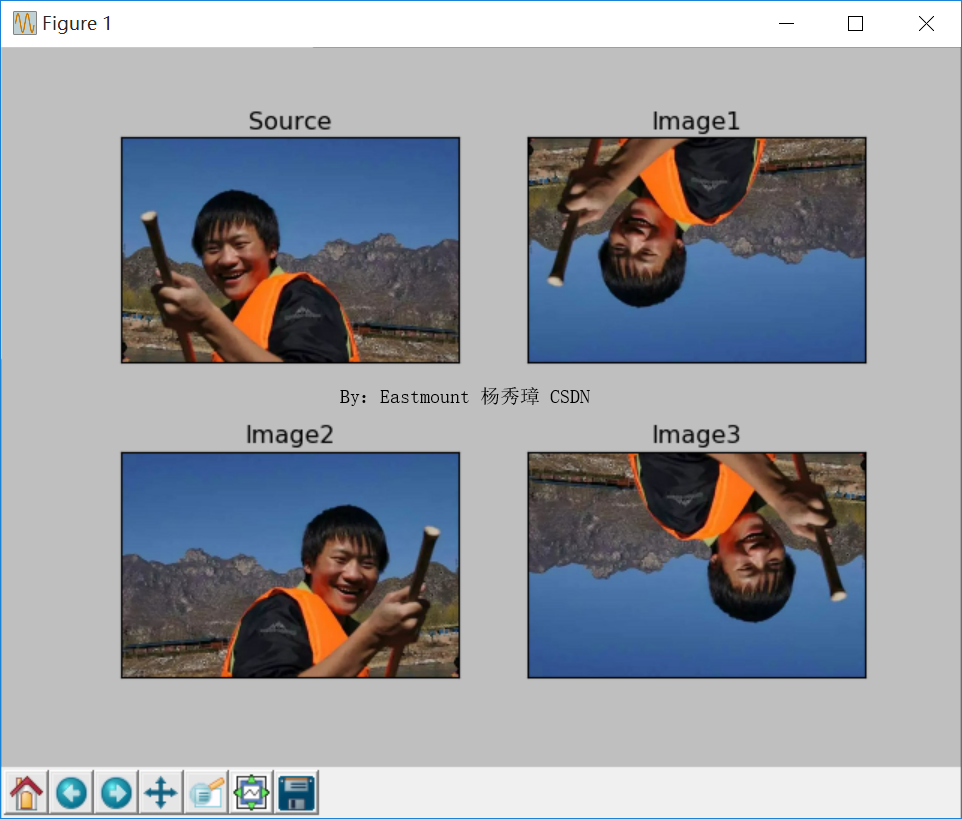四、图像平移
图像平移：设（x0, y0）是缩放后的坐标，（x, y）是缩放前的坐标，dx、dy为偏移量，则公式如下：
$\left[ \begin{matrix} x_0 & y_0 & 1 \end{matrix} \right] =\left[ \begin{matrix} x & y & 1 \end{matrix} \right] \left[ \begin{matrix} 1 & 0 & 0 \\ 0 & 1 & 0 \\ dx & dy & 1 \end{matrix} \right] \tag{3}$
图像平移首先定义平移矩阵M，再调用warpAffine()函数实现平移，核心函数如下：
M = np.float32([[1, 0, x], [0, 1, y]])
shifted = cv2.warpAffine(image, M, (image.shape, image.shape))
完整代码如下所示：
#encoding:utf-8
import cv2
import numpy as np
import matplotlib.pyplot as plt

#读取图片
image = cv2.cvtColor(img,cv2.COLOR_BGR2RGB)

#图像平移 下、上、右、左平移
M = np.float32([[1, 0, 0], [0, 1, 100]])
img1 = cv2.warpAffine(image, M, (image.shape, image.shape))

M = np.float32([[1, 0, 0], [0, 1, -100]])
img2 = cv2.warpAffine(image, M, (image.shape, image.shape))

M = np.float32([[1, 0, 100], [0, 1, 0]])
img3 = cv2.warpAffine(image, M, (image.shape, image.shape))

M = np.float32([[1, 0, -100], [0, 1, 0]])
img4 = cv2.warpAffine(image, M, (image.shape, image.shape))

#显示图形
titles = [ 'Image1', 'Image2', 'Image3', 'Image4']
images = [img1, img2, img3, img4]
for i in xrange(4):
plt.subplot(2,2,i+1), plt.imshow(images[i], 'gray')
plt.title(titles[i])
plt.xticks([]),plt.yticks([])
plt.show()

输出结果如下图所示：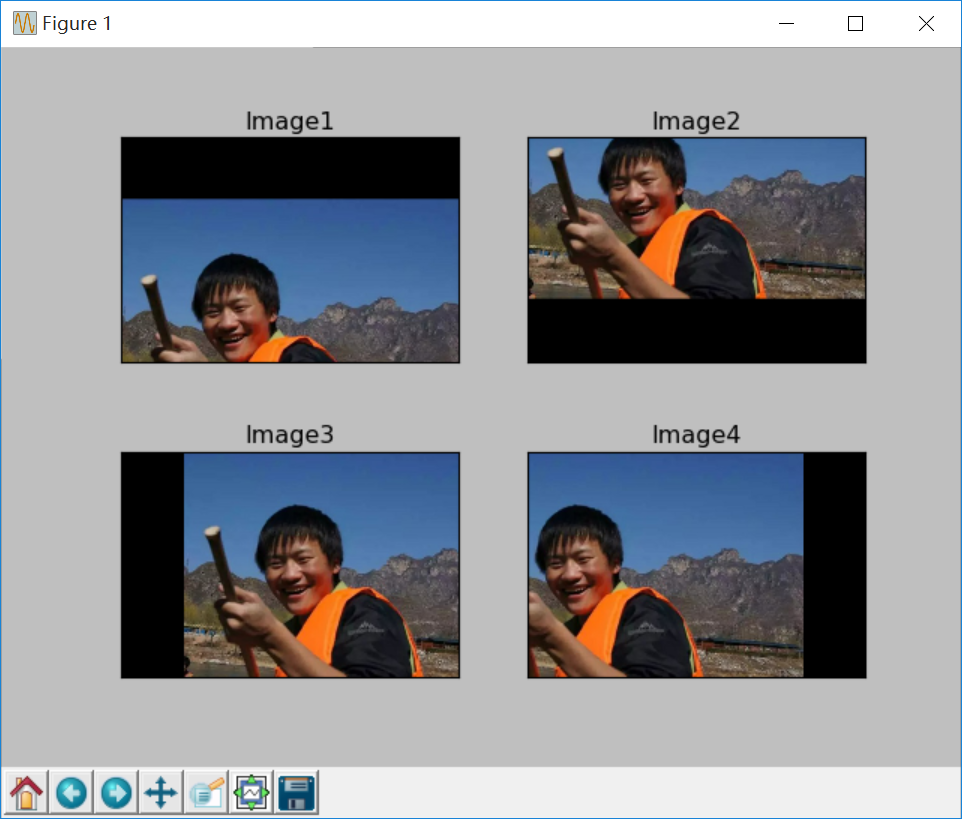三尺讲台，三寸舌，
三千桃李，三杆笔。
再累再苦，站在讲台前就是最美的自己，几个月的烦恼和忧愁都已消失，真的好享受这种状态，仿佛散着光芒，终于给低年级的同学上课了越早培养编程兴趣越好，恨不能倾囊相授。
即使当一辈子的教书匠，平平淡淡也喜欢，而且总感觉给学生讲课远不是课酬和职称所能比拟，这就是所谓的事业，所谓的爱好。
希望文章对大家有所帮助，如果有错误或不足之处，还请海涵。 准备出去休回家了，好好享受最美时光。
（By：Eastmount 2018-09-06 早10点 https://blog.csdn.net/Eastmount/）

2020年8月18新开的“娜璋AI安全之家”，主要围绕Python大数据分析、网络空间安全、人工智能、Web渗透及攻防技术进行讲解，同时分享CCF、SCI、南核北核论文的算法实现。娜璋之家会更加系统，并重构作者的所有文章，从零讲解Python和安全，写了近十年文章，真心想把自己所学所感所做分享出来，还请各位多多指教，真诚邀请您的关注！谢谢。展开全文Python 图像处理 OpenCV
• ## 图像旋转

千次阅读 2018-11-14 11:25:58
转自https://blog.csdn.net/shanghaiqianlun/article/details/52537485 1. 基本概念     1.1 基本公式 从图中我们可以得到如下关系： r = (X – X0)/cos(α) = (Y0-Y)/sin(α) Xn = r*cos(θ + α) +... ...
• 图像的选装，按照指定中心点逆时针旋转b角度，这个稍微有点麻烦 具体实现起来相比较而言有一点麻烦，不着急，一步一步来，首先需要将像素矩阵中第i行j列元素坐标(i,j)转化为坐标系坐标(x0,y0) 同理坐标系坐标转化...图像旋转 Win32API 图像处理
• 前面我们提到了图像的缩放变换，可以用矩阵乘法的形式来表达变换后的像素位置映射关系。 那么，对于旋转变换呢？我们可以同样将其想象成二维平面上矢量的旋转。如下图所示，矢量[x1,y1][x_1,y_1][x1​,y1​]逆时针...
• 前面我们提到了图像的缩放变换，可以用矩阵乘法的形式来表达变换后的像素位置映射关系。 那么，对于旋转变换呢？我们可以同样将其想象成二维平面上矢量的旋转。如下图所示，矢量[x1,y1]逆时针旋转θθθ度到了[x2,...
• 图像旋转就是将图像按一定角度旋转，依据当前点坐标计算出来的旋转后的坐标往不是整数，因此需要进行插值。常用的插值方法有最近邻插值法、线性插值法和样条插值法（这个我也不懂）。最近邻插值速度快，效果差；双向...
• ## 图像几何变换

千次阅读 2020-04-19 11:43:48
一，求点(x1, y1)关于点(x0, y0)旋转a度后的坐标 1、首先可以将问题简化，先算点(x1, y1)关于源点逆时针旋转a度后的坐标，求出之后加上x0，y0即可。 2、关于源点旋转，用极坐标表示 设x1 = Rcos(θ), y1 = Rsin...图像旋转
• 图形图像处理－之－任意角度的高质量的快速的图像旋转 上篇 纯软件的任意角度的快速旋转  HouSisong@GMail.com 2007.04.26   (2009.03.09 可以到这里下载旋转算法的完整的可以编译的项目源代码: ...
• ## 图像旋转算法原理-- 旋转矩阵

万次阅读 多人点赞 2011-09-05 19:58:19
图1     图2     图3      图4      图5      图6     图7     图8算法
• 话不多说上代码 filename3=imread('D:\My Documents\MATLAB\image\21.jpg'); I3=rgb2gray(filename3); figure imshow(I3) figure imshow(imrotate(I3,45,'nearest'))图像处理 matlab
• ## matlab实现图像旋转

万次阅读 2019-03-19 17:04:15
旋转后，原图像的四个角点应在新图像的四条边上（即新图像的大小是包含原图像旋转后的最小矩形）；旋转的角度可以是0~ 360度；函数的调用形式是J=myrotate(I,angle)；不允许使用imrotate。 原理 原理部分仅仅是...数字图像 matlab 图像旋转
• # -*- coding: utf-8 -*- from PIL import Image from numpy import * pil_im = Image.open('e:/aa/3.bmp') pil_im = pil_im.rotate(2) print pil_im.size pil_im.save('e:/aa/30.bmp')python 图像处理
• import numpy as np import cv2 img=cv2.imread("/home/lisa/dataset/HandDataSet_720_1280/chenshuyi/image/0.jpg",1) cv2.imshow("temp",img) cv2.waitKey(0) img90=np.rot90(img) cv2.imshow("rotate",img90)
• 有两个图，已经加载到Mat中，期中的一个图是另一个图经过一定的旋转得到的，我想利用OpenCV来获得被旋转图是旋转了多少度。希望各位大虾能给点思路或者方法，谢谢了！c++ opencv
• Halcon中实现旋转的方式由两种。一种是rotate_image，该方式实现简单，但只能绕中心旋转。二是affine_trans_image，该方式实现较复杂，但是可以实现绕任意位置的旋转python opencv